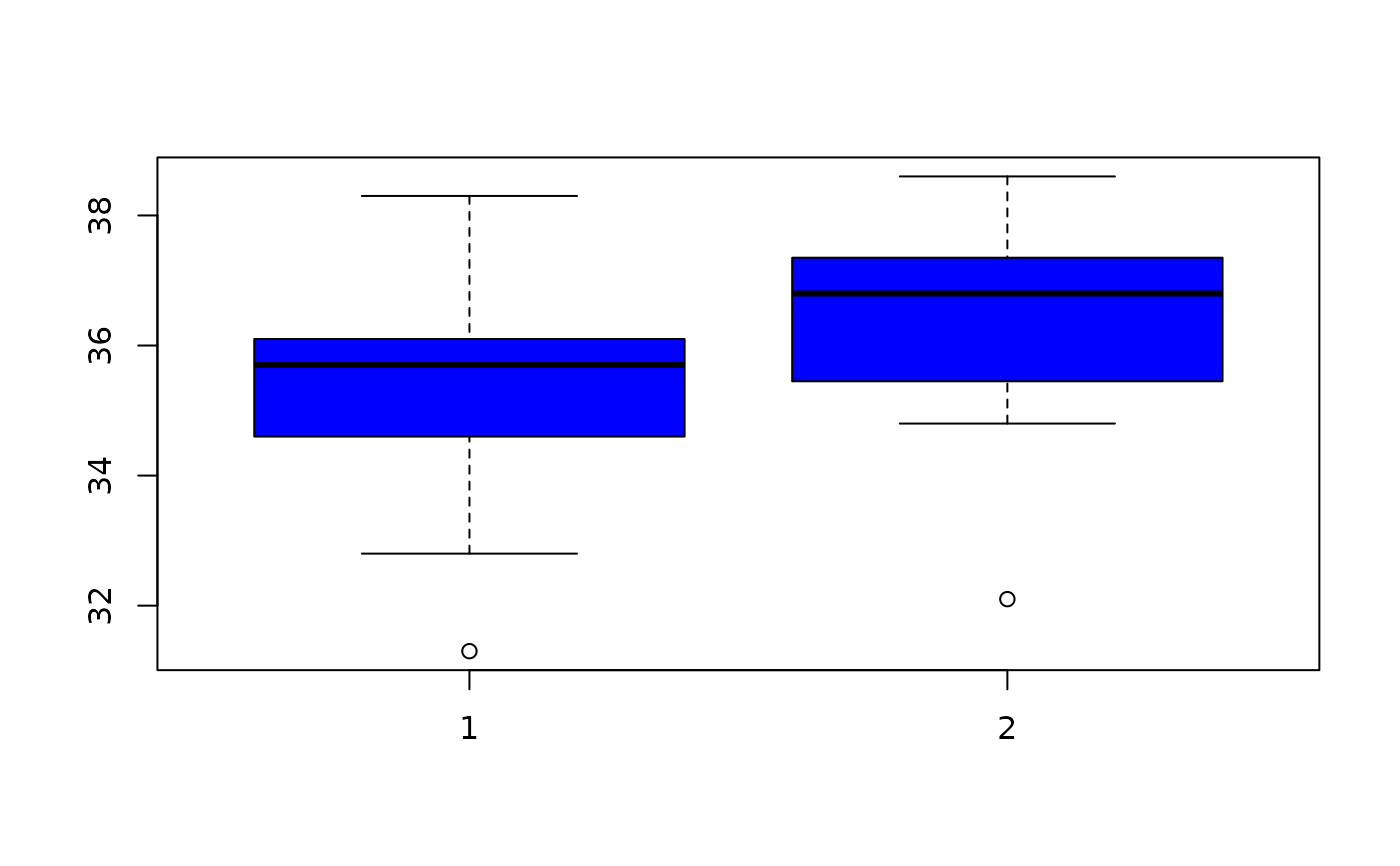Data for Exercise 7.44

Viscosit

## Format

A data frame/tibble with 11 observations on two variables

first

viscosity measurement for a certain substance on day one

second

viscosity measurement for a certain substance on day two

## Examples


boxplot(Viscosit$first, Viscosit$second, col = "blue")t.test(Viscosit$first, Viscosit$second, var.equal = TRUE)
#>
#> 	Two Sample t-test
#>
#> data:  Viscosit$first and Viscosit$second
#> t = -1.2578, df = 20, p-value = 0.2229
#> alternative hypothesis: true difference in means is not equal to 0
#> 95 percent confidence interval:
#>  -2.7067041  0.6703404
#> sample estimates:
#> mean of x mean of y
#>  35.28182  36.30000
#>